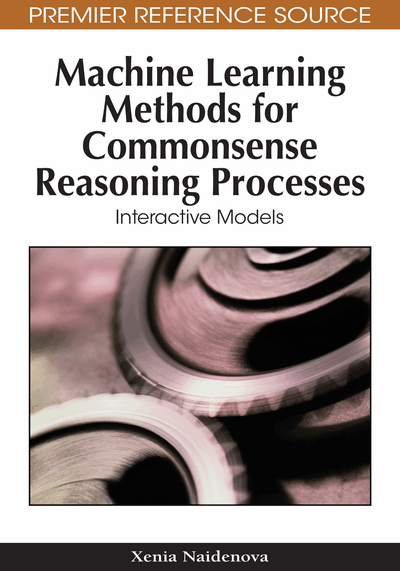# The Coordination of Commonsense Reasoning Operations

Xenia Naidenova (Military Medical Academy, Russia)
DOI: 10.4018/978-1-60566-810-9.ch003
Available
\$37.50
No Current Special Offers

## Abstract

In this chapter, a conception of commonsense reasoning is developed based on mutually coordinated operations on objects, classes of objects, and properties of objects. This conception goes back to the model of classification processes advanced and described in (Piaget, & Inhelder, 1959). The classification operations are the integral part of any reasoning about time, space, things, events, motions, etc. They consolidate all the forms of reasoning and make it possible to present knowledge as a system of interconnected relations.
Chapter Preview
Top

## Three Interrelated Algebraic Lattices Of Classes, Objects, And Properties

The principle “atom” of commonsense human reasoning is concept. The concepts are represented by the use of names. We shall consider the following roles of names in reasonings: a name can be the name of an object, the name of a class of objects and the name of a classification or collection of classes. With respect to the role of name in knowledge representation schemes, it can be the name of attribute and the name of attribute’s value. A class of objects may contain only one object, hence the name of object is a particular case of the name of class. For example, fir-tree can serve as the name of a tree or as the name of a class of trees. Each attribute genarates a classification of a given set of objects, hence the names of attributes can be considered as the names of classifications and the attribute values can be considered as the names of classes.. In the knowledge bases, the sets of names for objects, classes and classifications must not intersect.

Let k be the name of an objects’ class, let c be the name of a property of objects (the value of an attribute), and let o be the name of an object. Each class or property has only one maximal set of objects as its interpretation that is the set of objects belonging to this class or possessing this property: kI(k) = { o: ok}, cI(c) = { o: oc}, where the relation ‘≤’ denotes ‘is a’ relation and has causal nature (the dress is red, an apple is a fruit). Each object has only one corresponding set of all its properties: С(o) = { c: oc}. We shall say that C(o) is the description of object o. The link o → C(o) is also of causal nature. We shall say that C(k) = { ∩C(o): ok} is the description of class k, where C(k) is a collection of properties associated with each object of class k. The link kC(k) is also of causal nature. Figure 1 illustrates the causal links between classes of objects, properties of objects, and objects.

It is clear that each description (a set of properties) has one and only one interpretation (the set of objects possessing this set of properties). But the same set of objects can be the interpretation of different descriptions (equivalent with respect to their interpretations). The equivalent descriptions of the same class are to be said the different names of this class. The task of inferring the equivalence relations between names of classes and properties underlies the processes of commonsense reasoning.

The identity has the following logical content: class A is equivalent to property a (Aa) if and only if the interpretations I (A), I (a) on the set of conceivable objects are equal I (A) = I (a). It is possible to define also the relationship of approximate identity between concepts: a approximates B (aB) if and only if the relation I (a) ⊆ I (B) is satisfied. We can consider instead of one property (concept) any subset of properties joined by the union ∪ operation: (c1c2 ∪ … ci ∪…. ∪ cn) ≤ B.

## Complete Chapter List

Search this Book:
Reset